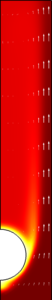# Application Gallery

## Heat Transfer by Free Convection

Application ID: 122

This example describes an array of heating tubes submerged in a vessel with fluid flow entering at the bottom.

This is a multiphysics model because it involves fluid dynamics coupled with heat transfer. The pressure and the velocity field are the solution of the Navier-Stokes equations, while the temperature is solved through the heat equation.

In this model, the equations are coupled in both directions. The buoyancy force lifting the fluid is entered in the compressible Navier-Stokes equations via a force term depending on the temperature through the density. At the same time, the heat equation accounts for convective heat transfer.

The implementation uses two physics interfaces: the Laminar Flow interface for laminar single-phase fluid flow and the Heat Transfer in Fluids interface for heat transfer. In addition, the multiphysics features Temperature Coupling and Flow Coupling are used to set automatically the coupling between the two interfaces.This model example illustrates applications of this type that would nominally be built using the following products: